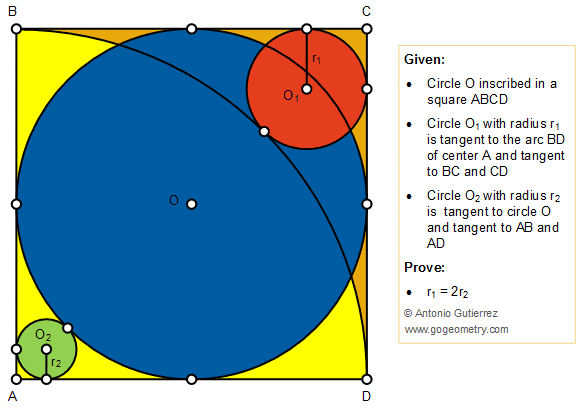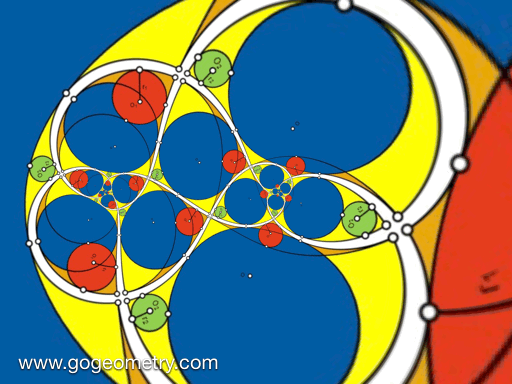Geometry Problem 1427: Circle inscribed in a square, Arc, Tangent Line, Tangent Circles, Radii

A circle O is inscribed in a square ABCD. As shown in the figure below, a circle O1 with radius r1 is tangent to the arc BD of center A and tangent to BC and CD. A circle O2 with radius r2 is tangent to circle O and tangent to AB and AD. Prove that r1 = 2r2.Geometric Art of Problem 1427: Sketching, Typography, iPad AppsAnimation of the Conformal Mapping or Transformation of Problem 1427Conformal Mapping

A conformal mapping or conformal transformation is a continuous mapping preserving the form of infinitesimal figures. This conformal map produces a realistic view of the original image or map. This the conformal transformation of problem1427